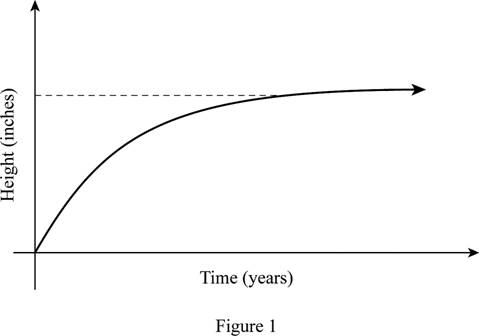# Whether the function which reflects a person’s height at age t is one-to-one.### Single Variable Calculus: Concepts...

4th Edition
James Stewart
Publisher: Cengage Learning
ISBN: 9781337687805### Single Variable Calculus: Concepts...

4th Edition
James Stewart
Publisher: Cengage Learning
ISBN: 9781337687805

#### Solutions

Chapter 1.6, Problem 14E
To determine

## Whether the function which reflects a person’s height at age t is one-to-one.

Expert Solution

The function is not a one-to one function.

### Explanation of Solution

Result used:

Horizontal line test states that the graph of the function is one-to-one function if and only if a horizontal line intersects the graph exactly once.

Calculation:

The given function is, f(t)=Height at age t.

It is known that the height of a person will remain constant after a certain age. That is the growth will be stopped and maintains a constant height for many years.

The graph of the function f(t)=Height at age t is shown below in Figure 1.From Figure 1, it is observed that the horizontal line intersects the curve at many points, which means it fails the horizontal line test.

Thus, it can be concluded that the function f(t)=Height at age t is not a one-to-one function.

### Have a homework question?

Subscribe to bartleby learn! Ask subject matter experts 30 homework questions each month. Plus, you’ll have access to millions of step-by-step textbook answers!